# 全球名校课程作业分享系列(6)--斯坦福计算机视觉与深度学习CS231n之神经网络细解与优化尝试

• To-Do：

• [x] 统一所有的数学符号和代码符号

• [x] 统一所有的术语名称

• [x] 术语的英文词汇对应

• [x] 线性模型的名字是？perceptron？

• [x] 损失函数的计算公式和符号是否严格和合适？

• [x] denominator layout？

• [ ] BN梯度的证明
• [x] ​ bn_param[‘running_mean’] = running_mean
​ bn_param[‘running_var’] = running_var

（我是盗图大仙，所有图片资源全部来源于网络，若侵权望告知～）

CS231n课程讲师Andrej Karpathy在他的博客上写过一篇文章Hacker’s guide to Neural Networks，其中的精神是我最欣赏的一种教程写作方式：“My exposition will center around code and physical intuitions instead of mathematical derivations. Basically, I will strive to present the algorithms in a way that I wish I had come across when I was starting out.”

“…everything became much clearer when I started writing code.”

## 待折腾的数据集## 关于神经网络，你起码应该知道的！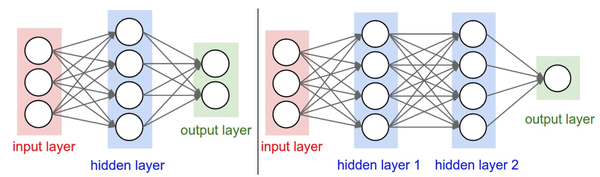## 所谓的前向传播

### 一个神经元的本事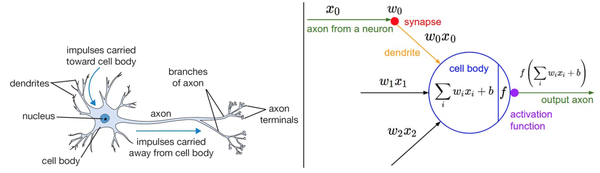$\sum _{i}{w}_{i}{x}_{i}+b={w}_{0}{x}_{0}+{w}_{1}{x}_{1}+\cdots +{w}_{D-1}{x}_{D-1}+b\phantom{\rule{0ex}{0ex}}$

$\underset{1×1}{\underset{⏟}{\left[\begin{array}{c}\sum _{i}{w}_{i}{x}_{i}+b\end{array}\right]}}=\underset{1×D}{\underset{⏟}{\left[\begin{array}{ccc}\cdots & {x}_{i}& \cdots \end{array}\right]}}\cdot \underset{D×1}{\underset{⏟}{\left[\begin{array}{c}⋮\\ {w}_{i}\\ ⋮\end{array}\right]}}+\underset{1×1}{\underset{⏟}{\left[\begin{array}{c}b\end{array}\right]}}$

$\underset{N×1}{\underset{⏟}{\left[\begin{array}{c}\sum _{i}{w}_{i}{x}_{i}+b\\ ⋮\end{array}\right]}}=\underset{N×D}{\underset{⏟}{\left[\begin{array}{ccc}\cdots & {x}_{i}& \cdots \\ & ⋮& \end{array}\right]}}\cdot \underset{D×1}{\underset{⏟}{\left[\begin{array}{c}⋮\\ {w}_{i}\\ ⋮\end{array}\right]}}+\underset{N×1}{\underset{⏟}{\left[\begin{array}{c}b\\ ⋮\\ ⋮\end{array}\right]}}$$f\left(x\right)=max\left(0,x\right)$

$\text{out}=f\left(\sum _{i}{w}_{i}{x}_{i}+b\right)=max\left(0,\sum _{i}{w}_{i}{x}_{i}+b\right)$

### 强大的层状神经元

$\underset{1×10}{\underset{⏟}{\left[\begin{array}{ccc}\sum _{i}{w}_{i}{x}_{i}+b& \cdots & \end{array}\right]}}=\underset{1×D}{\underset{⏟}{\left[\begin{array}{ccc}\cdots & {x}_{i}& \cdots \end{array}\right]}}\cdot \underset{D×10}{\underset{⏟}{\left[\begin{array}{ccc}⋮& & \\ {w}_{i}& \cdots & \cdots \\ ⋮& & \end{array}\right]}}+\underset{\underset{\text{Broadcasting}}{1×10}}{\underset{⏟}{\left[\begin{array}{ccc}b& \cdots & \end{array}\right]}}$，这说明$W$$W$$b$$b$的值没有训练好啊。那么究竟该如何训练出合适的参数呢？难道每次都要肉眼观察每个标签算出的得分再同时对比参数选的究竟好不好？再难道每次都要自己手调参数矩阵的每个值来观察得分效果么？当然不会这么傻啦，到时候一个叫损失函数的概念就登场了，且继续听故事先～

$\underset{1×10}{\underset{⏟}{\left[\begin{array}{ccc}\sum _{i}{w}_{i}{x}_{i}+b& \cdots & \end{array}\right]}}=\underset{1×D}{\underset{⏟}{\left[\begin{array}{ccc}\cdots & {x}_{i}& \cdots \end{array}\right]}}\cdot \underset{D×10}{\underset{⏟}{\left[\begin{array}{ccc}⋮& & \\ {w}_{i}& \cdots & \cdots \\ ⋮& & \end{array}\right]}}+\underset{\underset{\text{Broadcasting}}{1×10}}{\underset{⏟}{\left[\begin{array}{ccc}b& \cdots & \end{array}\right]}}$$\underset{N×M}{\underset{⏟}{\left[\begin{array}{ccc}& & \\ & max\left(0,{\stackrel{^}{x}}_{i}\right)& \\ & & \end{array}\right]}}\stackrel{←}{=}\underset{N×M}{\underset{⏟}{\left[\begin{array}{ccc}& & \\ & {\stackrel{^}{x}}_{i}& \\ & & \end{array}\right]}}\stackrel{←}{=}\underset{N×H}{\underset{⏟}{\left[\begin{array}{ccc}& & \\ & {x}_{i}& \\ & & \end{array}\right]}}\cdot \underset{H×M}{\underset{⏟}{\left[\begin{array}{ccc}& & \\ & {w}_{ij}& \\ & & \end{array}\right]}}+\underset{\underset{\text{Broadcasting}}{N×M}}{\underset{⏟}{\left[\begin{array}{ccc}& & \\ & {b}_{i}& \\ & & \end{array}\right]}}$

### 不废话了，看代码！

“…everything became much clearer when I started writing code.”

def affine_forward(x, w, b):
"""
Inputs:
- x: A numpy array containing input data, of shape (N, d_1, ..., d_k) 样本
- w: A numpy array of weights, of shape (D, M) 权重
- b: A numpy array of biases, of shape (M,) 偏置
Returns a tuple of:
- out: output, of shape (N, M)
- cache: (x, w, b)
"""
out = None      # 初始化
reshaped_x = np.reshape(x, (x.shape,-1))     # 确保x是一个规整的矩阵
out = reshape_x.dot(w) +b                       # out = w x +b
cache = (x, w, b)                               # 将该函数的输入值缓冲储存起来，以备后面计算梯度时使用
return out, cache

• 首先，需要对输入数据$x$$x$进行矩阵化，这是因为如果这代表的是第一层神经元前向传播，那么对于我们的图片数据集CIFAR-10，其每张图片输入进来的数据$x$$x$的shape是$\left(N,32,32,3\right)$$(N,32,32,3)$，是一个4维的array，所以需要将其reshape成$\left(N,3072\right)$$(N,3072)$的2维矩阵，其中每行是由一串3072个数字所代表的一个图片样本。np.reshape(x, (x.shape,-1)) 中的 -1 是指剩余可填充的维度，所以这段代码意思就是保证reshape后的矩阵行数是$N$$N$，剩余的维度信息都规则的排场一行即可。
• 输出的 cache 变量就是把该函数的输入值$\left(x,w,b\right)$$(x,w,b)$存为元组(tuple)再输出出去以备用，它当然不会流到下一层神经元，但其在后面会讲到的反向传播算法中利用到，由此可见我们是有多么的老谋深算啊！
• 接下来是重点： out = reshape_x.dot(w) +b 这句代码就表达了某一层神经元中，每个神经元可以并行的独立完成上两节提到的线性感知器模型，对每个图像给出自己的评价分数，与代码对应一致的内涵可见如下矩阵表达式：

$\underset{N×M}{\underset{⏟}{\left[\begin{array}{ccc}& & \\ & \text{out}& \\ & & \end{array}\right]}}=\underset{N×D}{\underset{⏟}{\left[\begin{array}{ccc}& & \\ & \text{reshape_x}& \\ & & \end{array}\right]}}\cdot \underset{D×M}{\underset{⏟}{\left[\begin{array}{ccc}& & \\ & \text{w}& \\ & & \end{array}\right]}}+\underset{\underset{\text{Broadcasting}}{N×M}}{\underset{⏟}{\left[\begin{array}{ccc}& & \\ & \text{b}& \\ & & \end{array}\right]}}$

A      (4d array):  8 x 1 x 6 x 1
B      (3d array):      7 x 1 x 5
Result (4d array):  8 x 7 x 6 x 5

$>↓\left[\begin{array}{ccc}>\cdots & b& \cdots \\ >\cdots & b& \cdots \\ >\cdots & b& \cdots >\end{array}\right]\right\}N行>$

def relu_forward(x):
"""
Computes the forward pass for a layer of rectified linear units (ReLUs).
Input:
- x: Inputs, of any shape
Returns a tuple of:
- out: Output, of the same shape as x
- cache: x
"""
out = np.maximum(0, x)   # 取x中每个元素和0做比较
cache = x                # 缓冲输入进来的x矩阵
return out, cache

def affine_relu_forward(x, w, b):
"""
Convenience layer that perorms an affine transform followed by a ReLU

Inputs:
- x: Input to the affine layer
- w, b: Weights for the affine layer

Returns a tuple of:
- out: Output from the ReLU
- cache: Object to give to the backward pass
"""
a, fc_cache = affine_forward(x, w, b) # 线性模型
out, relu_cache = relu_forward(a)   # 激活函数
cache = (fc_cache, relu_cache)  # 缓冲的是元组：(x, w, b, (a))
return out, cache## 传说中的反向传播### 审判官！损失函数登场！

def softmax_loss(z, y):
"""
Computes the loss and gradient for softmax classification.
Inputs:
- z: Input data, of shape (N, C) where z[i, j] is the score for the jth class
for the ith input.
- y: Vector of labels, of shape (N,) where y[i] is the label for x[i] and
0 <= y[i] < C
Returns a tuple of:
- loss: Scalar giving the loss
- dz: Gradient of the loss with respect to z
"""
probs = np.exp(z - np.max(z, axis=1, keepdims=True))    # 1
probs /= np.sum(probs, axis=1, keepdims=True)           # 2
N = z.shape                                          # 3
loss = -np.sum(np.log(probs[np.arange(N), y])) / N      # 4
dz = probs.copy()
dz[np.arange(N), y] -= 1
dz /= N
return loss, dz

• softmax_loss(z, y) 函数的输入数据是shape为(N, C)的矩阵z和shape为(N, )的一维array行向量y。由于损失函数的输入数据来自神经网络的输出层，所以这里的矩阵z中的N代表是数据集样本图片的个数，C代表的是数据集的标签个数，对应于CIFAR-10的训练集来说，z矩阵的shape应该为(50000, 10)，其中矩阵元素数值就是CIFAR-10的训练集数据经过整个神经网络层到达输出层，对每一张样本图片(每行)打分，给出对应各个标签(每列)的得分分数。一维array行向量y内的元素数值储存的是训练样本图片数据源的正确标签，数值范围是 $0⩽{y}_{i}$0\leqslant y_i，亦即 ${y}_{i}=0,1,\dots ,9$$y_i=0,1,…,9$
• 前2行代码定义了probs变量。首先，np.max(z, axis=1, keepdims=True) 是对输入矩阵x在横向方向挑出一个最大值，并要求保持横向的维度输出一个矩阵，即输出为一个shape为(N, 1)的矩阵，其每行的数值表示每张样本图片得分最高的标签对应得分；然后，再 np.exp(z - ..) 的操作表示的是对输入矩阵z的每张样本图片的所有标签得分都被减去该样本图片的最高得分，换句话说，将每行中的数值进行平移，使得最大值为0；再接下来对所有得分取exp函数，然后在每个样本图片中除以该样本图片中各标签的总和(np.sum)，最终得到一个与矩阵z同shape的(N, C)矩阵probs。上述得到矩阵probs中元素数值的过程对应的就是softmax函数

${S}_{ij}\equiv \frac{{e}^{{z}_{ij}}}{\sum _{j}{e}^{{z}_{ij}}}=\frac{C{e}^{{z}_{ij}}}{C\sum _{j}{e}^{{z}_{ij}}}=\frac{{e}^{{z}_{ij}+\mathrm{log}C}}{\sum _{j}{e}^{{z}_{ij}+\mathrm{log}C}}\equiv \text{probs}$

$$z_{ij} \equiv \underbrace{\begin{bmatrix} 1 & 1 & 1 & 2 \ 2 & 2 & 2 & 3 \ 3 & 3 & 3 & 5 \end{bmatrix}}_{3\times 4} \overset{-\max}{\Longrightarrow } \underbrace{ $\left[\begin{array}{cccc}-1& -1& -1& 0\\ -1& -1& -1& 0\\ -2& -2& -2& 0\end{array}\right]$ }_{3\times 4} \overset{\exp}{\Longrightarrow } \underbrace{ $\left[\begin{array}{cccc}0.368& 0.368& 0.368& 1\\ 0.368& 0.368& 0.368& 1\\ 0.135& 0.135& 0.135& 1\end{array}\right]$ }_{3\times 4} \ \overset{1/\text{sum}}{\Longrightarrow } \underbrace{ $\left[\begin{array}{cccc}0.175& 0.175& 0.175& 1/2.104\\ 0.175& 0.175& 0.175& 1/2.104\\ 0.096& 0.096& 0.096& 1/1.405\end{array}\right]$ }_{3\times 4} \equiv \text{probs}_{ij}$$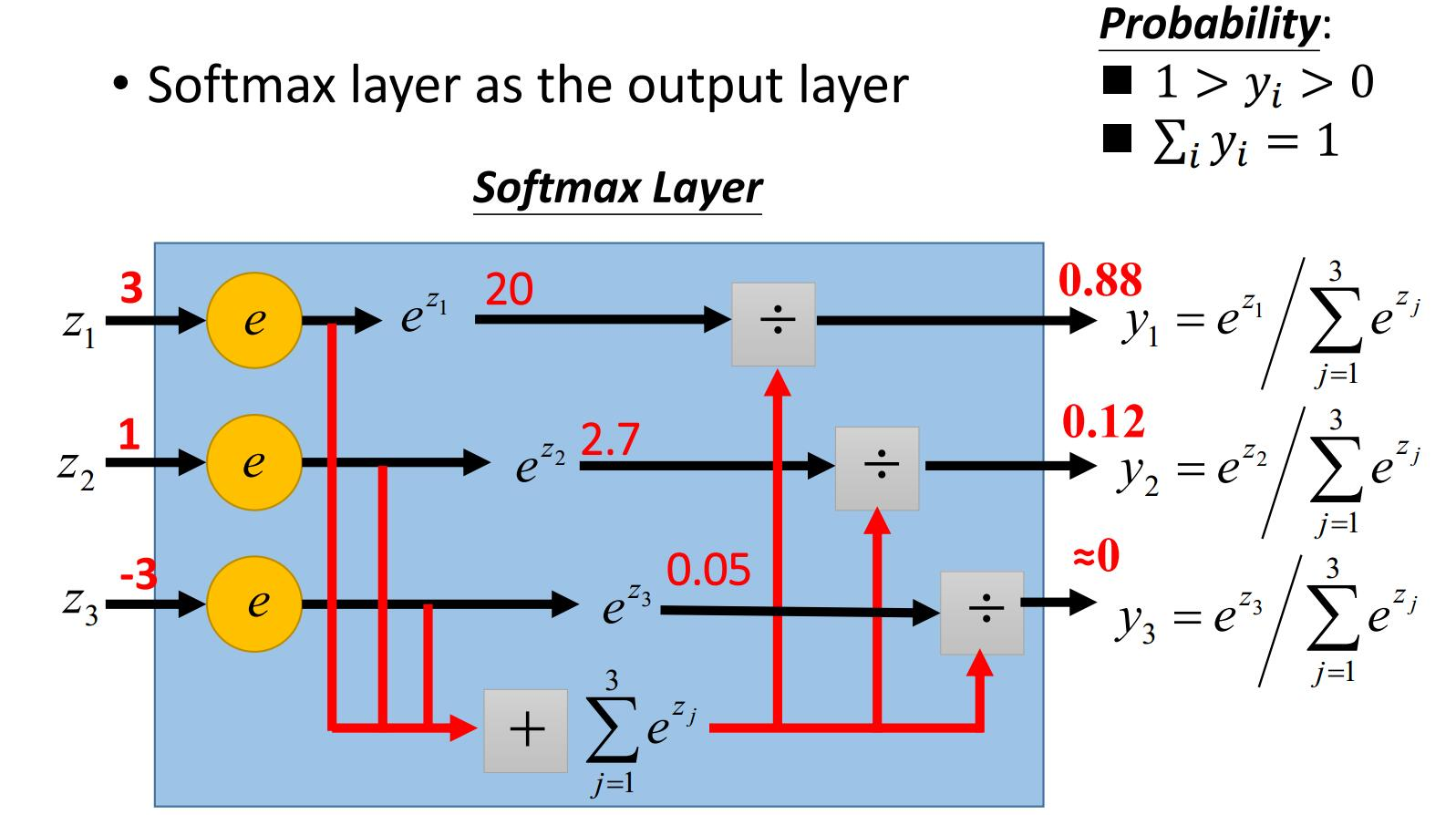• 定义损失函数： loss = -np.sum(np.log(probs[np.arange(N), y])) / N，输出是一个scalar数 loss。其数学含义是这样的

$L\equiv \sum _{i;j={y}_{i}}{L}_{ij}=-\frac{1}{N}\sum _{i;j={y}_{i}}\mathrm{log}\left({S}_{ij}\right)=-\frac{1}{N}\sum _{i;j={y}_{i}}\mathrm{log}\left(\frac{{e}^{{z}_{ij}}}{\sum _{j}{e}^{{z}_{ij}}}\right)$

$\begin{array}{rl}\begin{array}{c}{S}_{ij}={\text{probs}}_{ij}\equiv \underset{3×4}{\underset{⏟}{\left[\begin{array}{cccc}0.175& 0.175& 0.175& 1/2.104\\ 0.175& 0.175& 0.175& 1/2.104\\ 0.096& 0.096& 0.096& 1/1.405\end{array}\right]}}\\ {y}_{i}\equiv \underset{1×3}{\underset{⏟}{\left[\begin{array}{ccc}2& 0& 1\end{array}\right]}}\end{array}\right\}\stackrel{\text{probs[np.arange(N), y]}}{⟹}& \underset{1×3}{\underset{⏟}{\left[\begin{array}{ccc}0.175& 0.096& 0.175\end{array}\right]}}\\ \stackrel{\mathrm{log}}{⟹}& \underset{1×3}{\underset{⏟}{\left[\begin{array}{ccc}-1.743& -2.343& -1.743\end{array}\right]}}\\ \stackrel{\frac{-1}{N}\text{sum}}{⟹}& -\frac{1}{3}\left(-1.743-2.343-1.743\right)⇒L\end{array}$

### 跟着梯度走！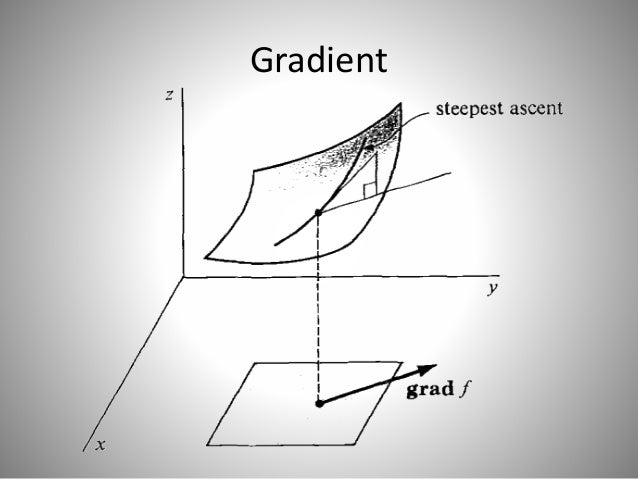def softmax_loss(z, y):
"""
Computes the loss and gradient for softmax classification.
Inputs:
- z: Input data, of shape (N, C) where z[i, j] is the score for the jth class
for the ith input.
- y: Vector of labels, of shape (N,) where y[i] is the label for x[i] and
0 <= y[i] < C
Returns a tuple of:
- loss: Scalar giving the loss
- dz: Gradient of the loss with respect to z, of shape (N, C)
"""
probs = np.exp(z - np.max(z, axis=1, keepdims=True))
probs /= np.sum(probs, axis=1, keepdims=True)
N = z.shape
loss = -np.sum(np.log(probs[np.arange(N), y])) / N
dz = probs.copy()           # 1 probs.copy() 表示获得变量probs的副本
dz[np.arange(N), y] -= 1    # 2
dz /= N                     # 3
return loss, dz

• 这里的后三行计算出的 dz 变量是损失函数关于从输出层输入来的数据矩阵z的梯度，其shape与数据矩阵z相同，即(N, C)。其严格的数学解析定义(证明过程)是：

\left.\begin{matrix} \begin{align*} &\frac{\partial L_{ij}}{\partial z_{ij}} = \frac{1}{N}(S_{ij}-1),,,\ &\frac{\partial L_{ij}}{\partial z_{il}} = \frac{1}{N}S_{il},,,,,,,(l\neq j) \end{align*} \end{matrix}\right} \Rightarrow dL\equiv # \sum_{i;j=y_i}dL_{ij} \sum_i\Big[\frac{1}{N}(S_{iy_j}-1)dz_{iy_i} +\frac{1}{N}S_{il}dz_{il} \Big],,,,,(l\neq y_i)

\begin{align*} \left.\begin{matrix} S_{ij} \equiv \underbrace{ $\left[\begin{array}{cccc}0.175& 0.175& 0.175& 1/2.104\\ 0.175& 0.175& 0.175& 1/2.104\\ 0.096& 0.096& 0.096& 1/1.405\end{array}\right]$ }_{3\times 4} \ y_{i}\equiv \underbrace{ }_{1\times 3} \end{matrix}\right} & \overset{dx[\text{np.arange(N), y}] -= 1}{\Longrightarrow } \underbrace{ }_{3\times 4} \ & \overset{\frac{1}{N}}{\Longrightarrow } \frac{1}{3} \underbrace{ }_{3\times 4} =\text{dx}\equiv\Big[\frac{\partial L_{ij}}{\partial x_{il}}\Big] \end{align*}

$$\Big[\frac{\partial L_{ij}}{\partial z_{il}}\Big] \Leftrightarrow \underbrace{\begin{bmatrix} \frac{\partial L_{0 0}}{\partial z_{00}} & \cdots & {\color{Red}{\frac{\partial L_{0 y_i}}{\partial z_{0y_i}}}} &\cdots & \cdots\ {\color{Red}{\frac{\partial L_{1y_0}}{\partial z_{1y_0}}}} & \cdots &\frac{\partial L_{1 j}}{\partial z_{1j}} & \cdots & \cdots \ \cdots & {\color{Red}{\frac{\partial L_{iy_i}}{\partial z_{y_i}}}} & \cdots & \frac{\partial L_{ij}}{\partial z_{ij}} &\cdots\ \cdots &\cdots &\cdots & \cdots &\cdots \end{bmatrix}}_{N\times C} \Leftrightarrow \frac{1}{N} \underbrace{ }_{N\times C} \Rightarrow \text{dz}$$

### 链式的反向传播！def affine_backward(dout, cache):
"""
Computes the backward pass for an affine layer.
Inputs:
- dout: Upstream derivative, of shape (N, M)    上一层的散度输出
- cache: Tuple of:
- z: Input data, of shape (N, d_1, ... d_k)
- w: Weights, of shape (D, M)
- b: biases, of shape (M,)
Returns a tuple of:
- dz: Gradient with respect to z, of shape (N, d1, ..., d_k)
- dw: Gradient with respect to w, of shape (D, M)
- db: Gradient with respect to b, of shape (M,)
"""
z, w, b = cache
dz, dw, db = None, None, None
reshaped_x = np.reshape(z, (z.shape, -1))
dz = np.reshape(dout.dot(w.T), z.shape)    # np.dot() 是矩阵乘法
dw = (reshaped_x.T).dot(dout)
db = np.sum(dout, axis=0)
return dz, dw, db

• 我们定义 affine_backward(dout, cache) = (dz, dw, db) 函数来描述输出层神经元的反向传播。其中shape为(N, M)的 dout 矩阵就是损失函数 $L$ 关于该层在 affine_forward() 函数正向输出数据 out 的梯度，其对应于我们上一节定义的 softmax_loss() 函数输出的 dz 矩阵。梯度在输出层反向传播的话，M的大小就等于样本图片的标签数，即 $M=10$cache 元组是正向流入输出层的神经元的数据x和输出层的参数(w, b)，即有 z, w, b = cache，其中输出层的输入数据z是未经过reshaped的一个多维array，shape为$(N,d_1,…,d_k)$，权重矩阵W的shape是(D, M)，偏置b的shape是(M, )。

“矩阵维度匹配”到底是什么意思？这其实是个挺”猥琐”的方法，故事是这样的：

\begin{align*} \underbrace{\begin{bmatrix} & & \ & \text{dx} & \ & & \end{bmatrix}}_{N\times 32\times32\times3} \overset{\text{np.reshape}}{\Longleftarrow } \underbrace{ }_{N\times D} &= \underbrace{ }_{N\times M} \cdot {\color{blue} { \underbrace{ }_{M\times D} }}\ \underbrace{ }_{D\times M} &= {\color{blue} { \underbrace{ }_{D\times N} }} \cdot \underbrace{ }_{N\times M} \ \underbrace{ }_{1\times M} & = {\color{blue} { \underbrace{ }_{1\times N} }} \cdot \underbrace{ }_{N\times M} \end{align*}

dx = np.reshape(dout.dot(w.T), x.shape)
dw = (reshaped_x.T).dot(dout)
db = np.sum(dout, axis=0)

• 可以看到对矩阵w和矩阵reshaped_x的转置操作，以及与dout矩阵的矩阵乘法前后顺序的安排，都是考虑到梯度矩阵(dx, dw, db)维数满足约定的前提下，所得到唯一可能的梯度矩阵表达形式。
• 代码中 np.reshape(.., x.shape) 要求输出的矩阵与多维array输入数据x的shape同型。
• 代码中 np.sum(.., axis=0) 表示每列元素求和，显然这个矩阵操作等价于我们在上面写的矩阵表达式哈！

  def relu_backward(dout, cache):
"""
Computes the backward pass for a layer of rectified linear units (ReLUs).
Input:
- dout: Upstream derivatives, of any shape
- cache: Input x, of same shape as dout
Returns:
- dx: Gradient with respect to x
"""
dx, x = None, cache
dx = (x > 0) * dout
# 与所有x中元素为正的位置处，位置对应于dout矩阵的元素保留，其他都取0
return dx

def affine_relu_backward(dout, cache):
"""
Backward pass for the affine-relu convenience layer
"""
fc_cache, relu_cache = cache            # fc_cache = (x, w, b)   relu_cache = a
da = relu_backward(dout, relu_cache)    # da = (x > 0) * relu_cache
dx, dw, db = affine_backward(da, fc_cache)
return dx, dw, db

• 代码 dx = (x > 0) * dout ，表示损失函数关于在ReLU激活函数处流入流出数据的梯度的反向传递。下图是某层神经元激活函数部分中的数据正反向传播的示意图：

\begin{align*} \underbrace{\begin{bmatrix} {\color{green} {-1 }}& {\color{green} {-1 }} & 3\ {\color{green} {-2}} & (\text{x}) & 4 \ 2 & 4 & {\color{green} {-5 }} \end{bmatrix}}_{N\times H} & \overset{\text{forward}}{\Longrightarrow } \underbrace{ }_{N\times H} \ \underbrace{ }_{N\times H} & \overset{\text{backward}}{\Longleftarrow } \underbrace{ }_{N\times H} \end{align*}

### 小结：拉起神经网络的大网！class TwoLayerNet(object): # 我们的2层全连接神经网络
"""
首先，需要初始化我们的神经网络。
毕竟，数据从输入层第一次流入到神经网络里，我们的参数(W,B)不能为空，
也不能都太大或太小，因为参数(W,B)的初始化是相当重要的，
对整个神经网络的训练影响巨大，但如何proper的初始化参数仍然没有定论
目前仍有很多paper在专门讨论这个话题。
"""
def __init__(self
,input_dim=3*32*32     # 每张样本图片的数据维度大小
,hidden_dim=100        # 隐藏层的神经元个数
,num_classes=10        # 样本图片的分类类别个数是
,weight_scale=1e-3):   # 初始化参数的权重尺度(标准偏差)
"""
我们把需要学习的参数(W,B)都存在self.params字典中，
其中每个元素都是都是numpy arrays:
"""
self.params = {}
"""
我们用标准差为weight_scale的高斯分布初始化参数W,
偏置B的初始都为0:
(其中randn函数是基于零均值和标准差的一个高斯分布)
"""
self.params["W1"] = weight_scale * np.random.randn(input_dim
,hidden_dim)
self.params["b1"] = np.zeros((hidden_dim,))
self.params["W2"] = weight_scale * np.random.randn(hidden_dim
,num_classes)
self.params["b2"] = np.zeros((num_classes,))
"""
可以看到,
隐藏层的参数矩阵行数是3*32*32，列数是100；
输出层的参数矩阵行数是100，列数10.
"""

# 接下来，我们最后定义一个loss函数就可以完成神经网络的构造
def loss(self, X, y):
"""
首先，输入的数据X是一个多维的array，shape为(样本图片的个数N*3*32*32),
y是与输入数据X对应的正确标签，shape为(N,)。
其中存有loss关于隐藏层和输出层的参数(W,B)的梯度值：
"""

# 数据X在隐藏层和输出层的前向传播：
h1_out, h1_cache = affine_relu_forward(X
,self.params["W1"]
,self.params["b1"])
scores, out_cache = affine_forward(h1_out
,self.params["W2"]
,self.params["b2"])

# 输出层后，结合正确标签y得出损失值和其在输出层的梯度：
loss, dout = softmax_loss(scores, y)

# 损失值loss的梯度在输出层和隐藏层的反向传播：
dout, dw2, db2 = affine_backward(dout, out_cache)
_, dw1, db1 = affine_relu_backward(dout, h1_cache)

"""
可以看到图片样本的数据梯度dout只起到了带路的作用，
最终会舍弃掉，我们只要loss关于参数的梯度，
"""
return loss, grads

NO~ no~ no~

## 高速运转的加强版神经网络

。。。

### 论自我惩罚后的救赎之路——正则化

“背答案”的后果可是很严重的，必须要接受惩罚！loss += 0.5 * self.reg * (np.sum(self.params["W1"] ** 2) + \
np.sum(self.params["W2"] ** 2))
dW2 += self.reg * self.params["W2"]
dW1 += self.reg * self.params["W1"]

• self.reg 是正则化强度，这不是一个要优化的学习参数，需要在训练之前设置好，取其值为0就意味着不考虑正则化。
• np.sum(self.params["W1"] ** 2) 表示对参数矩阵 $w_1$ 中的每一个元素都取平方，并全部加起来得到一个scalar数。

### 批量归一化def batchnorm_forward(x, gamma, beta, bn_param):
"""
During training the sample mean and (uncorrected) sample variance are
computed from minibatch statistics and used to normalize the incoming data.
During training we also keep an exponentially decaying running mean of the mean
and variance of each feature, and these averages are used to normalize data
t test-time.

At each timestep we update the running averages for mean and variance using
an exponential decay based on the momentum parameter:

running_mean = momentum * running_mean + (1 - momentum) * sample_mean
running_var = momentum * running_var + (1 - momentum) * sample_var

Note that the batch normalization paper suggests a different test-time
behavior: they compute sample mean and variance for each feature using a
large number of training images rather than using a running average. For
this implementation we have chosen to use running averages instead since
they do not require an additional estimation step; the torch7 implementation
of batch normalization also uses running averages.

Input:
- x: Data of shape (N, D)
- gamma: Scale parameter of shape (D,)
- beta: Shift paremeter of shape (D,)
- bn_param: Dictionary with the following keys:
- mode: 'train' or 'test'; required
- eps: Constant for numeric stability
- momentum: Constant for running mean / variance.
- running_mean: Array of shape (D,) giving running mean of features
- running_var Array of shape (D,) giving running variance of features

Returns a tuple of:
- out: of shape (N, D)
- cache: A tuple of values needed in the backward pass
"""
mode = bn_param['mode']
eps = bn_param.get('eps', 1e-5)
momentum = bn_param.get('momentum', 0.9)

N, D = x.shape
running_mean = bn_param.get('running_mean', np.zeros(D, dtype=x.dtype))
running_var = bn_param.get('running_var', np.zeros(D, dtype=x.dtype))

out, cache = None, None
if mode == 'train':     # 训练模式
sample_mean = np.mean(x, axis=0)    # 矩阵x每一列的平均值 shaple: (D,)
sample_var = np.var(x, axis=0)      # 矩阵x每一列的方差 shape: (D,)
x_hat = (x - sample_mean) / (np.sqrt(sample_var + eps))
out = gamma * x_hat + beta
cache = (x, sample_mean, sample_var, x_hat, eps, gamma, beta)
running_mean = momentum * running_mean + (1 - momentum) * sample_mean
running_var = momentum * running_var + (1 - momentum) * sample_var

elif mode == 'test':    # 测试模式
out = (x - running_mean) * gamma / (np.sqrt(running_var + eps)) + beta
else:
raise ValueError('Invalid forward batchnorm mode "%s"' % mode)

# Store the updated running means back into bn_param
bn_param['running_mean'] = running_mean
bn_param['running_var'] = running_var

return out, cache

• batchnorm_forward() 函数的输入矩阵x可以看做是输入的样本数据(或者是经过线性函数的输出值)，shape为(N, D)，N是样本图片的个数，D是特征个数(本例中就是像素点数)。参数(gamma, beta)是我们新的待优化学习的参数，shape都为(D, )，这样一来，我们的神经网络模型参数增加到四个(w, b, gamma, beta)。
• 输入进来的 bn_param 是BN算法的参数字典，其中存有 eps 数值变量精度。 是为了避免分母除数为0的情况所使用的微小正数。mode 存好了当前神经网络的状态。我们在之前的故事中所谈过的数据正向流动和损失函数梯度的反向传播都已经默认了是 train 模式，因为我们最终的目标是希望根据样本图片训练我们的神经网络模型(中的参数)，使得它进入 test 模式下时，输入没有正确标签的样本图片可以给出正确的标签。参数字典中 momentum 参数是最优化中的”超参数”常数，我们会在最后说明其中的内涵，在这里只要知道它会默认取值为0.9就好了。running_mean, running_var即移动平均值和移动方差值，会在train阶段随着样本的输入不断变化，
• train 训练模式下，sample_meansample_var 分别是对x的每一列算得平均值和标准差向量，shape都为(D, )。若这是第一隐藏层中神经元的BN层，这相当于是把每张样本图像对应像素维度看作结构化数据的一个”特征”(共3072个)，然后算出所有样本图片里每个像素特征下的平均值和标准差。
• 接下来就是重点了，我们要操作的数学公式是这样的：• 在代码中，x_hat = (x - sample_mean) / (np.sqrt(sample_var + eps))out = gamma * x_hat + beta ，仍可以明显的看到Broadcasting机制的应用。参数向量(gamma, beta) 都被broadcasting拉成与矩阵x相同shape(N, D)的矩阵，然后在每一个对应元素上做如数学公式后两行所示的运算。在公式中 eps 的存在是必要的，因为数据某一列的标准差 sample_var 为0是可能的，比如仅一张图片流入神经网络的时候。
• train 模式里，running_mean, running_var 会在每经过一个BN层进行一次迭代计算～并在离开每一个BN层之前保存替换掉原BN参数字典中的 running_mean, runing_var 值。在训练结束后，这两个参数将会用于 test 测试模式下的每一个BN层中。其实你若留意英文注释的话，就会明白上面代码中的操作并不是BN算法的作者原始的处理方式，其本意是由于神经网络一旦训练完毕，参数都会基本固定下来，这个时候即使是每批样本图片再进入我们的神经网络，那么BN层计算的平均值和标准差都是固定不变的，所以我们可以采用这些数值来作为测试样本所需要的均值、标准差。然而，我们上述代码中所采用的估计整个训练集的方法，会高效简洁得多，严格地说，这叫一次指数平滑法(Single exponential smoothing)

def affine_bn_relu_forward(x, w, b, gamma, beta, bn_param):
"""
Inputs:
- x: Array of shape (N, D1); input to the affine layer
- w, b: Arrays of shape (D2, D2) and (D2,) giving the weight and bias for
the affine transform.
- gamma, beta: Arrays of shape (D2,) and (D2,) giving scale and shift
parameters for batch normalization.
- bn_param: Dictionary of parameters for batch normalization.
Returns:
- out: Output from ReLU, of shape (N, D2)
- cache: Object to give to the backward pass.
"""
a, fc_cache = affine_forward(x, w, b)
a_bn, bn_cache = batchnorm_forward(a, gamma, beta, bn_param) #BN层，注意！它在ReLU层前
out, relu_cache = relu_forward(a_bn)    # ReLU层
cache = (fc_cache, bn_cache, relu_cache)
return out, cachedef batchnorm_backward(dout, cache):
"""
Inputs:
- dout: Upstream derivatives, of shape (N, D)
- cache: Variable of intermediates from batchnorm_forward.
Returns a tuple of:
- dx: Gradient with respect to inputs x, of shape (N, D)
- dgamma: Gradient with respect to scale parameter gamma, of shape (D,)
- dbeta: Gradient with respect to shift parameter beta, of shape (D,)
"""
x, mean, var, x_hat, eps, gamma, beta = cache
N = x.shape
dgamma = np.sum(dout * x_hat, axis=0)   # 第5行公式
dbeta = np.sum(dout * 1.0, axis=0)      # 第6行公式
dx_hat = dout * gamma                   # 第1行公式
dx_hat_numerator = dx_hat / np.sqrt(var + eps)      # 第3行第1项(未负求和)
dx_hat_denominator = np.sum(dx_hat * (x - mean), axis=0)    # 第2行前半部分
dx_1 = dx_hat_numerator                 # 第4行第1项
dvar = -0.5 * ((var + eps) ** (-1.5)) * dx_hat_denominator  # 第2行公式
# Note var is also a function of mean
dmean = -1.0 * np.sum(dx_hat_numerator, axis=0) + \
dvar * np.mean(-2.0 * (x - mean), axis=0)  # 第3行公式(部分)
dx_var = dvar * 2.0 / N * (x - mean)    # 第4行第2项
dx_mean = dmean * 1.0 / N               # 第4行第3项
# with shape (D,), no trouble with broadcast
dx = dx_1 + dx_var + dx_mean            # 第4行公式

return dx, dgamma, dbeta

• 代码中已经给出了详细的与数学公式对应的注释。
• 提前提个醒，在 test 模式下，我们并不需要有反向传播这一步骤，只需要样本图片数据经过神经网络后，在输出层给出的得分即可。

def affine_bn_relu_backward(dout, cache):
"""
Backward pass for the affine-batchnorm-relu convenience layer.
"""
fc_cache, bn_cache, relu_cache = cache
da_bn = relu_backward(dout, relu_cache)     # ReLU层
da, dgamma, dbeta = batchnorm_backward(da_bn, bn_cache) # BN层，反向传播时在ReLU后
dx, dw, db = affine_backward(da, fc_cache)
return dx, dw, db, dgamma, dbeta

### 就是这么任性！——Dropout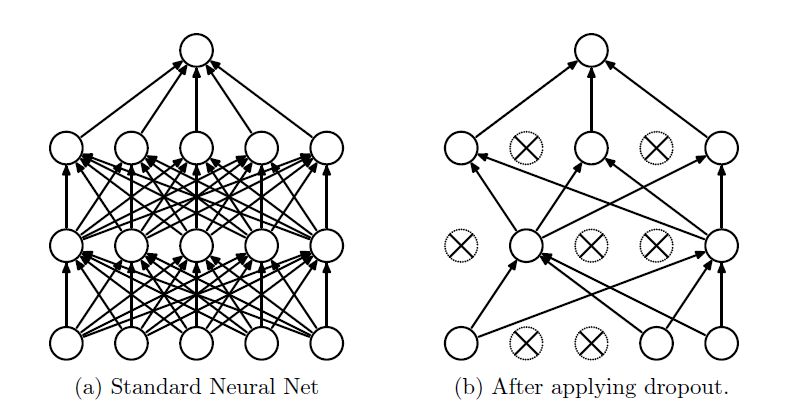def dropout_forward(x, dropout_param):
"""
Performs the forward pass for (inverted) dropout.
Inputs:
- x: Input data, of any shape
- dropout_param: A dictionary with the following keys:
- p: Dropout parameter. We drop each neuron output with probability p.
- mode: 'test' or 'train'. If the mode is train, then perform dropout;
if the mode is test, then just return the input.
- seed: Seed for the random number generator. Passing seed makes this
function deterministic, which is needed for gradient checking but not in
real networks.
Outputs:
- out: Array of the same shape as x.
- cache: A tuple (dropout_param, mask). In training mode, mask is the dropout
mask that was used to multiply the input; in test mode, mask is None.
"""
p, mode = dropout_param['p'], dropout_param['mode']
if 'seed' in dropout_param:
np.random.seed(dropout_param['seed'])

out = None
# 训练模式
if mode == 'train':
keep_prob = 1 - p
mask = (np.random.rand(*x.shape) < keep_prob) / keep_prob
# 测试模式
elif mode == 'test':
out = x

out = out.astype(x.dtype, copy=False)

return out, cache

• 输入数据矩阵x，其shape可以任意。dropout的参数字典 dropout_param 中存有超参数 pmode，分别代表每层神经元们被失活的概率和当前 dropout 层是训练模式，还是测试模式。该参数字典中的可以有随机数生成种子 seed，要来标记随机性。

• 在训练模式下，首先，代码 (np.random.rand(*x.shape)，表示根据输入数据矩阵x，亦即经过”激活”后的得分，生成一个相同shape的随机矩阵，其为均匀分布的随机样本[0,1)。然后将其与可被保留神经元的概率 keep_prob 做比较，就可以得到一个随机真值表作为随机失活遮罩(mask)。原始的办法是：由于在训练模式时，我们丢掉了部分的激活值，数值调整 out = mask * x 后造成整体分布的期望值的下降，因此在预测时就需要乘上一个概率 1/keep_prob，才能保持分布的统一。不过，我们用一种叫做inverted dropout的技巧，就是如上面代码所示，直接在训练模式下多除以一个概率 keep_prob，那么在测试模式下就不用做任何操作了，直接让数据通过dropout层即可。如下图所示：• 在最后，函数输出经过dropout且与输入x相同shape的out输出矩阵和缓冲保留dropout的参数字典 dropout_param，其中含有该层的失活概率超参数 p ，模式状态 mode，和随机失活遮罩 mask，以在反向传播中备用。

def dropout_backward(dout, cache):
"""
Perform the backward pass for (inverted) dropout.
Inputs:
- dout: Upstream derivatives, of any shape
- cache: (dropout_param, mask) from dropout_forward.
"""
mode = dropout_param['mode']

dx = None
if mode == 'train':

elif mode == 'test':
dx = dout
return dx

• 梯度反向传播时使用同样的 mask将被遮罩的梯度置零。

Regularization of Neural Networks using DropConnect

### 构造任意深度的神经网络！

class FullyConnectedNet(object):
"""
一个任意隐藏层数和神经元数的全连接神经网络，其中 ReLU 激活函数，sofmax 损失函数，同时可选的
采用 dropout 和 batch normalization(批量归一化)。那么，对于一个L层的神经网络来说，其
框架是：

{affine - [batch norm] - relu - [dropout]} x (L - 1) - affine - softmax

其中的[batch norm]和[dropout]是可选非必须的，框架中{...}部分将会重复L-1次，代表
L-1 个隐藏层。

与我们在上面的故事中定义的 TwoLayerNet() 类保持一致，所有待学习的参数都会存在
self.params 字典中，并且最终会被最优化 Solver() 类训练学习得到(后面的故事会谈到)。
"""
"""
"""
#1# 第一步是初始化我们的 FullyConnectedNet() 类：
def __init__(self
,hidden_dims       # 一个列表，元素个数是隐藏层数，元素值为该层神经元数
,input_dim=3*32*32 # 默认输入神经元的个数是3072个(匹配CIFAR-10数据集)
,num_classes=10    # 默认输出神经元的个数是10个(匹配CIFAR-10数据集)
,dropout=0         # 默认不开启dropout，若取(0,1)表示失活概率
,use_batchnorm=False   # 默认不开启批量归一化，若开启取True
,reg=0.0           # 默认无L2正则化，取某scalar表示正则化的强度
,weight_scale=1e-2 # 默认0.01，表示权重参数初始化的标准差
,dtype=np.float64  # 默认np.float64精度，要求所有的计算都应该在此精度下。
,seed=None):       # 默认无随机种子，若有会传递给dropout层。
"""
"""
# 实例(Instance)中增加变量并赋予初值，以方便后面的 loss() 函数调用：
self.use_batchnorm = use_batchnorm
self.use_dropout = dropout > 0  # 可见，若dropout若为0时，为False
self.reg = reg
self.num_layers = 1 + len(hidden_dims)  # 在loss()函数里，
# 我们用神经网络的层数来标记规模
self.dtype = dtype
self.params = {}                # self.params 空字典保存待训练学习的参数
"""
"""
# 定义所有隐藏层的参数到字典 self.params 中：
in_dim = input_dim  # Eg: in_dim = D
for i, h_dim in enumerate(hidden_dims): # Eg:(i, h_dim)=(0, H1)、(1, H2)...
# Eg: W1(D, H1)、W2(H1, H2)...           小随机数为初始值
self.params["W%d" % (i+1,)] = weight_scale * \
np.random.randn(in_dim
,h_dim)
# Eg: b1(H1,)、b2(H2,)...                0为初始值
self.params["b%d" % (i+1,)] = np.zeros((h_dim,))
if use_batchnorm:                   # 若有批量归一化层
# Eg: gamma1(H1,)、gamma2(H2,)...    1为初始值
# Eg: beta1(H1,)、beta2(H2)...       0为初始值
self.params["gamma%d" % (i+1,)] = np.ones((h_dim,))
self.params["beta%d" % (i+1,)] = np.zeros((h_dim,))
in_dim = h_dim  # 将该隐藏层的列数传递给下一层的行数
"""
"""
# 定义输出层的参数到字典 params 中：
self.params["W%d" % (self.num_layers,)] = weight_scale * \
np.random.randn(in_dim
,num_classes)
self.params["b%d" % (self.num_layers,)] = np.zeros((num_classes,))
"""
"""
"""
当开启 dropout 时，我们需要在每一个神经元层中传递一个相同的
dropout 参数字典 self.dropout_param ，以保证每一层的神经元们
都知晓失活概率p和当前神经网络的模式状态mode(训练／测试)。
"""
self.dropout_param = {} # dropout的参数字典
if self.use_dropout:    # 如果use_dropout的值是(0,1)，即启用dropout
# 设置mode默认为训练模式，取p为失活概率
self.dropout_param = {'mode': 'train', 'p': dropout}
if seed is not None:    # 如果有seed随机种子，存入seed
self.dropout_param['seed'] = seed
"""
"""
"""
当开启批量归一化时，我们要定义一个BN算法的参数列表 self.bn_params ，
以用来跟踪记录每一层的平均值和标准差。其中，第0个元素 self.bn_params
表示前向传播第1个BN层的参数，第1个元素 self.bn_params 表示前向传播
第2个BN层的参数，以此类推。
"""
self.bn_params = []     # BN算法的参数列表
if self.use_batchnorm:  # 如果开启批量归一化，设置每层mode默认为训练模式
self.bn_params = [{'mode': 'train'} for i in range(self.num_layers - 1)]
# 上面 self.bn_params 列表的元素个数是hidden layers的个数
"""
"""
# 最后，调整所有的待学习神经网络参数为指定计算精度：np.float64
for k, v in self.params.items():
self.params[k] = v.astype(dtype)
"""
"""
#2# 第二步是定义我们的损失函数：
def loss(self, X, y=None):
"""
和 TwoLayerNet() 一样：
首先，输入的数据X是一个多维的array，shape为(样本图片的个数N*3*32*32),
y是与输入数据X对应的正确标签，shape为(N,)。
# 在训练模式下：#
其中存有loss关于隐藏层和输出层的参数(W,B,gamma,beta)的梯度值.
# 在测试模式下：#
我们的loss函数只需要直接给出输出层后的得分即可。
"""
"""
"""
# 把输入数据源矩阵X的精度调整一下
X = X.astype(self.dtype)
# 根据正确标签y是否为None来调整模式是test还是train
mode = 'test' if y is None else 'train'
"""
"""
"""
确定了当前神经网络所处的模式状态后，
就可以设置 dropout 的参数字典和 BN 算法的参数列表中的mode了，
因为他们在不同的模式下行为是不同的。
"""
if self.dropout_param is not None:  # 如果开启dropout
self.dropout_param['mode'] = mode
if self.use_batchnorm:              # 如果开启批量归一化
for bn_param in self.bn_params:
bn_param['mode'] = mode
"""
"""
scores = None
"""
"""
"""
%前向传播%
如果开启了dropout，我们需要将dropout的参数字典 self.dropout_param
在每一个dropout层中传递。
如果开启了批量归一化，我们需要指定BN算法的参数列表 self.bn_params
对应前向传播第一层的参数，self.bn_params对应第二层的参数，以此类推。
"""
fc_mix_cache = {}       # 初始化每层前向传播的缓冲字典
if self.use_dropout:    # 如果开启了dropout，初始化其对应的缓冲字典
dp_cache = {}
"""
"""
# 从第一个隐藏层开始循环每一个隐藏层，传递数据out，保存每一层的缓冲cache
out = X
for i in range(self.num_layers - 1):    # 在每个hidden层中循环
w, b = self.params["W%d" % (i + 1,)], self.params["b%d" % (i + 1,)]
if self.use_batchnorm:              # 若开启批量归一化
gamma = self.params["gamma%d" % (i + 1,)]
beta = self.params["beta%d" % (i + 1,)]
out, fc_mix_cache[i] = affine_bn_relu_forward(out, w, b
,gamma
,beta
,self.bn_params[i])
else:                               # 若未开启批量归一化
out, fc_mix_cache[i] = affine_relu_forward(out, w, b)
if self.use_dropout:                # 若开启dropout
out, dp_cache[i] = dropout_forward(out, self.dropout_param)
# 最后的输出层
w = self.params["W%d" % (self.num_layers,)]
b = self.params["b%d" % (self.num_layers,)]
out, out_cache = affine_forward(out, w, b)
scores = out
"""
可以看到，上面对隐藏层的每次循环中，out变量实现了自我迭代更新；
fc_mix_cache 缓冲字典中顺序地存储了每个隐藏层的得分情况和模型参数(其中可内含BN层)；
dp_cache 缓冲字典中单独顺序地保存了每个dropout层的失活概率和遮罩；
out_cache 变量缓存了输出层处的信息；
值得留意的是，若开启批量归一化的话，BN层的参数列表 self.bn_params[i]，
从第一层开始多出'running_mean'和'running_var'的键值保存在参数列表的每一个元素中，
形如：[{'mode': 'train','running_mean': ***,'running_var':***},{...}]
"""
"""
"""
# 接下来开始让loss函数区分不同模式：
if mode == 'test':  # 若是测试模式，输出scores表示预测的每个分类概率后，函数停止跳出。
return scores
"""
"""
"""
%反向传播%
既然运行到了这里，说明我们的神经网络是在训练模式下了，
接下来我们要计算损失值，并且通过反向传播，计算损失函数关于模型参数的梯度！
"""
loss, dout = softmax_loss(scores, y)
loss += 0.5 * self.reg * np.sum(self.params["W%d" % (self.num_layers,)]**2)
"""
你可能奇怪上面的loss损失值是不是有问题，还有其他隐藏层的权重矩阵的正则化呢？
别着急，我们要loss损失值的求解，跟随梯度的反向传播一点一点的算出来～
"""
dout, dw, db = affine_backward(dout, out_cache)
grads["W%d" % (self.num_layers,)] = dw + self.reg * \
self.params["W%d" % (self.num_layers,)]
for i in range(self.num_layers - 1):
ri = self.num_layers - 2 - i    # 倒数第ri+1隐藏层
loss += 0.5 * self.reg * \      # 迭代地补上每层的正则项给loss
np.sum(self.params["W%d" % (ri+1,)]**2)
if self.use_dropout:            # 若开启dropout
dout = dropout_backward(dout, dp_cache[ri])
if self.use_batchnorm:          # 若开启批量归一化
dout, dw, db, dgamma, dbeta = affine_bn_relu_backward(dout,
fc_mix_cache[ri])
else:                           # 若未开启批量归一化
dout, dw, db = affine_relu_backward(dout, fc_mix_cache[ri])
grads["W%d" % (ri+1,)] = dw + self.reg * self.params["W%d" % (ri+1,)]

return loss, grads  # 输出训练模式下的损失值和损失函数的梯度！## 最终章！

• 神经网络究竟是如何工作的呢？
• 如何才能更高效的工作？
• 神经网络框架是搭建了，但它是静态不动的，所以训练的动态细节是什么？

### 漫漫优化路——SGD with momentum

def sgd(w, dw, config=None):
"""
config format:
- learning_rate: Scalar learning rate.
"""
if config is None: config = {}
config.setdefault('learning_rate', 1e-2)

w -= config['learning_rate'] * dw
return w, config

• 函数的输入w, dw，分别是样本图片经过神经网络时的某个模型参数和该参数对应的损失函数梯度；config 如果没有在函数中输入的话，会默认为一个字典：{'learning_rate', 1e-2}，其中 learning_rate 通常叫做学习率，它是一个固定的常量，是一个超参数，即与“模型参数”不同，是需要人工经验在神经网络外部设置调整的，与待学习的模型参数不同。当神经网络在整个图片数据集上进行计算时，只要学习率足够低，总是能在损失函数上得到非负的进展。
• w -= config['learning_rate'] * dw 就是随机梯度下降的算法核心了，只需要在每一次参数更新时，减去以学习率为倍数的梯度值即可，最后输出更新后的新模型参数 w 和sgd算法的设置字典 configdef sgd_momentum(w, dw, config=None):
"""
Performs stochastic gradient descent with momentum.
config format:
- learning_rate: Scalar learning rate.
- momentum: Scalar between 0 and 1 giving the momentum value.
Setting momentum = 0 reduces to sgd.
- velocity: A numpy array of the same shape as w and dw used to store a
"""
if config is None: config = {}
config.setdefault('learning_rate', 1e-2)
config.setdefault('momentum', 0.9)
v = config.get('velocity', np.zeros_like(w))
next_w = None

v = config["momentum"] * v - config["learning_rate"] * dw
next_w = w + v
config['velocity'] = v

return next_w, config

• 函数的输入(w, dw)和输出(next_w, config)sgd() 函数格式以及含义完全对应一致，只不过 sgd_momentum() 函数的最优化超参数有三个 {'learning_rate': le-2, 'momentum': 0.9, 'velocity': np.zeros_like(w)}，其中的velocity 并不是经验可调的超参数，其对应于我们每一次更新时的“动量”，并且在每一次模型参数更新后，都会将更新后的“动量”再保存回 config 字典中。

An Overview on Optimization Algorithms in Deep Learning (I)

Advanced tutorials - momentum, activation function, etc

An overview of gradient descent optimization algorithms

### 夜黑风高，小试牛刀！

# Load the (preprocessed) CIFAR10 data.
data = get_CIFAR10_data()
for k, v in data.items():
print('%s: ' % k, v.shape)
"""
y_train:  (49000,)
y_test:  (1000,)
X_val:  (1000, 3, 32, 32)
y_val:  (1000,)
X_test:  (1000, 3, 32, 32)
X_train:  (49000, 3, 32, 32)
"""

import numpy as np
# 我们把优化算法的函数 sgd() 和 sgd_momentum() 定义在当前目录中的 optim.py 文件中
import optim

class Solver(object):
"""
我们定义的这个Solver类将会根据我们的神经网络模型框架——FullyConnectedNet()类，
在数据源的训练集部分和验证集部分中，训练我们的模型，并且通过周期性的检查准确率的方式，
以避免过拟合。

在这个类中，包括__init__()，共定义5个函数，其中只有train()函数是最重要的。调用
它后，会自动启动神经网络模型优化程序。

训练结束后，经过更新在验证集上优化后的模型参数会保存在model.params中。此外，损失值的
历史训练信息会保存在solver.loss_history中，还有solver.train_acc_history和
solver.val_acc_history中会分别保存训练集和验证集在每一次epoch时的模型准确率。
===============================
下面是给出一个Solver类使用的实例：
data = {
'X_train': # training data
'y_train': # training labels
'X_val': # validation data
'   y_val': # validation labels
}   # 以字典的形式存入训练集和验证集的数据和标签
model = FullyConnectedNet(hidden_size=100, reg=10) # 我们的神经网络模型
solver = Solver(model, data,            # 模型／数据
update_rule='sgd',        # 优化算法
optim_config={            # 该优化算法的参数
'learning_rate': 1e-3,  # 学习率
},
lr_decay=0.95,            # 学习率的衰减速率
num_epochs=10,            # 训练模型的遍数
batch_size=100,           # 每次丢入模型训练的图片数目
print_every=100)
solver.train()
===============================
# 神经网络模型中必须要有两个函数方法：模型参数model.params和损失函数model.loss(X, y)
A Solver works on a model object that must conform to the following API:
- model.params must be a dictionary mapping string parameter names to numpy
arrays containing parameter values. #
- model.loss(X, y) must be a function that computes training-time loss and
gradients, and test-time classification scores, with the following inputs
and outputs:
Inputs:     # 全局的输入变量
- X: Array giving a minibatch of input data of shape (N, d_1, ..., d_k)
- y: Array of labels, of shape (N,) giving labels for X where y[i] is the
label for X[i].
Returns:    # 全局的输出变量
# 用标签y的存在与否标记训练mode还是测试mode
If y is None, run a test-time forward pass and return: #
- scores: Array of shape (N, C) giving classification scores for X where
scores[i, c] gives the score of class c for X[i].
If y is not None, run a training time forward and backward pass and return
a tuple of:
- loss: Scalar giving the loss  # 损失函数值
- grads: Dictionary with the same keys as self.params mapping parameter
names to gradients of the loss with respect to those parameters.# 模型梯度
"""
"""
"""
#1# 初始化我们的 Slover() 类：
def __init__(self, model, data, **kwargs):
"""
Construct a new Solver instance.
# 必须要输入的函数参数：模型和数据
Required arguments:
- model: A model object conforming to the API described above
- data: A dictionary of training and validation data with the following:
'X_train': Array of shape (N_train, d_1, ..., d_k) giving training images
'X_val': Array of shape (N_val, d_1, ..., d_k) giving validation images
'y_train': Array of shape (N_train,) giving labels for training images
'y_val': Array of shape (N_val,) giving labels for validation images
# 可选的输入参数：
Optional arguments:
# 优化算法：默认为sgd
- update_rule: A string giving the name of an update rule in optim.py.
Default is 'sgd'.
# 设置优化算法的超参数：
- optim_config: A dictionary containing hyperparameters that will be
passed to the chosen update rule. Each update rule requires different
hyperparameters (see optim.py) but all update rules require a
'learning_rate' parameter so that should always be present.
# 学习率在每次epoch时衰减率
- lr_decay: A scalar for learning rate decay; after each epoch the learning
rate is multiplied by this value.
# 在训练时，模型输入层接收样本图片的大小，默认100
- batch_size: Size of minibatches used to compute loss and gradient during
training.
# 在训练时，让神经网络模型一次全套训练的遍数
- num_epochs: The number of epochs to run for during training.
# 在训练时，打印损失值的迭代次数
- print_every: Integer; training losses will be printed every print_every
iterations.
# 是否在训练时输出中间过程
- verbose: Boolean; if set to false then no output will be printed during
training.
"""
# 实例(Instance)中增加变量并赋予初值，以方便后面的 train() 函数等调用：
self.model = model                          # 模型
self.X_train = data["X_train"]              # 训练样本图片数据
self.y_train = data["y_train"]              # 训练样本图片的标签
self.X_val, self.y_val = data["X_val"], data["y_val"]   # 验证样本图片的数据和标签
"""
以下是可选择输入的类参数，逐渐一个一个剪切打包kwargs参数列表
"""
self.update_rule = kwargs.pop('update_rule', 'sgd') # 默认优化算法sgd
self.optim_config = kwargs.pop('optim_config', {})  # 默认设置优化算法为空字典
self.lr_decay = kwargs.pop('lr_decay', 1.0)         # 默认学习率不衰减
self.batch_size = kwargs.pop('batch_size', 100)     # 默认输入层神经元数100
self.num_epochs = kwargs.pop('num_epochs', 10)      # 默认神经网络训练10遍
#
self.print_every = kwargs.pop('print_every', 10)
self.verbose = kwargs.pop('verbose', True)          # 默认打印训练的中间过程
"""
异常处理：如果kwargs参数列表中除了上述元素外还有其他的就报错！
"""
if len(kwargs) > 0:
extra = ', '.join('"%s"' % k for k in kwargs.keys())
raise ValueError('Unrecognized arguments %s' % extra)
"""
异常处理：如果kwargs参数列表中没有优化算法，就报错！
将self.update_rule转化为优化算法的函数，即:
self.update_rule(w, dw, config) = (next_w, config)
"""
if not hasattr(optim, self.update_rule): # 若optim.py中没有写好的优化算法对应
raise ValueError('Invalid update_rule "%s"' % self.update_rule)
self.update_rule = getattr(optim, self.update_rule)
# 执行_reset()函数：
self._reset()
"""
"""
#2# 定义我们的 _reset() 函数，其仅在类初始化函数 __init__() 中调用
def _reset(self):
"""
重置一些用于记录优化的变量
"""
# Set up some variables for book-keeping
self.epoch = 0
self.best_val_acc = 0
self.best_params = {}
self.loss_history = []
self.train_acc_history = []
self.val_acc_history = []
# Make a deep copy of the optim_config for each parameter
self.optim_configs = {}
for p in self.model.params:
d = {k: v for k, v in self.optim_config.items()}
self.optim_configs[p] = d
""" 上面根据模型中待学习的参数，创建了新的优化字典self.optim_configs，
形如：{'b': {'learnning_rate': 0.0005}
,'w': {'learnning_rate': 0.0005}}，为每个模型参数指定了相同的超参数。
"""
"""
"""
#3# 定义我们的 _step() 函数，其仅在 train() 函数中调用
def _step(self):
"""
训练模式下，样本图片数据的一次正向和反向传播，并且更新模型参数一次。
"""
# Make a minibatch of training data # 输入数据准备
num_train = self.X_train.shape   # 要训练的数据集总数
"""
"""
# Compute loss and gradient     # 数据通过神经网络后得到损失值和梯度字典
self.loss_history.append(loss)  # 把本次算得的损失值记录下来
"""
"""
# Perform a parameter update    # 执行一次模型参数的更新
for p, w in self.model.params.items():
config = self.optim_configs[p]          # 取出模型参数p对应的优化超参数
next_w, next_config = self.update_rule(w, dw, config) # 优化算法
self.model.params[p] = next_w           # 新参数替换掉旧的
self.optim_configs[p] = next_config     # 新超参数替换掉旧的，如动量v
"""
"""
#4# 定义我们的 check_accuracy() 函数，其仅在 train() 函数中调用
def check_accuracy(self, X, y, num_samples=None, batch_size=100):
"""
根据某图片样本数据，计算某与之对应的标签的准确率
Inputs:
- X: Array of data, of shape (N, d_1, ..., d_k)
- y: Array of labels, of shape (N,)
- num_samples: If not None, subsample the data and only test the model
on num_samples datapoints.
- batch_size: Split X and y into batches of this size to avoid using too
much memory.

Returns:
- acc: Scalar giving the fraction of instances that were correctly
classified by the model.
"""

# Maybe subsample the data
N = X.shape                                  # 样本图片X的总数
if num_samples is not None and N > num_samples:
N = num_samples

# Compute predictions in batches
num_batches = N // batch_size
if N % batch_size != 0:
num_batches += 1
y_pred = []
for i in range(num_batches):
start = i * batch_size
end = (i + 1) * batch_size
scores = self.model.loss(X[start:end])
y_pred.append(np.argmax(scores, axis=1))
y_pred = np.hstack(y_pred)
acc = np.mean(y_pred == y)

return acc

#5# 定义我们最重要的 train() 函数
def train(self):
"""
首先要确定下来总共要进行的迭代的次数num_iterations，
"""
num_train = self.X_train.shape   # 全部要用来训练的样本图片总数
iterations_per_epoch = max(num_train // self.batch_size, 1) # 每遍迭代的次数
num_iterations = self.num_epochs * iterations_per_epoch # 总迭代次数
"""
开始迭代循环！
"""
for t in range(num_iterations):
self._step()
"""
上面完成了一次神经网络的迭代。此时，模型的参数已经更新过一次，
并且在self.loss_history中添加了一个新的loss值
"""
# Maybe print training loss 从self.loss_history中取最新的loss值
if self.verbose and t % self.print_every == 0:
print('(Iteration %d / %d) loss: %f' % (t + 1,
num_iterations, self.loss_history[-1]))
"""
"""
# At the end of every epoch, increment the epoch counter and
# decay the learning rate.
epoch_end = (t + 1) % iterations_per_epoch == 0
if epoch_end:   # 只有当t = iterations_per_epoch-1 时为True
self.epoch += 1 # 第一遍之后开始，从0自加1为每遍计数
for k in self.optim_configs: # 第一遍之后开始，每遍给学习率自乘一个衰减率
self.optim_configs[k]['learning_rate'] *= self.lr_decay
"""
"""
# Check train and val accuracy on the first iteration, the last
# iteration, and at the end of each epoch.
first_it = (t == 0)                 # 起始的t
last_it = (t == num_iterations - 1) # 最后的t
if first_it or last_it or epoch_end:    # 在最开始／最后／每遍结束时
train_acc = self.check_accuracy(self.X_train, self.y_train,
num_samples=1000) # 随机取1000个训练图看准确率
val_acc = self.check_accuracy(self.X_val, self.y_val)
self.train_acc_history.append(train_acc)  # 计算全部验证图片的准确率
self.val_acc_history.append(val_acc)
"""
"""
if self.verbose:    # 在最开始／最后／每遍结束时，打印准确率等信息
print('(Epoch %d / %d) train acc: %f; val_acc: %f' % (
self.epoch, self.num_epochs, train_acc, val_acc))
"""
"""
# 在最开始／最后／每遍结束时，比较当前验证集的准确率和过往最佳验证集
# Keep track of the best model
if val_acc > self.best_val_acc:
self.best_val_acc = val_acc
self.best_params = {}
for k, v in self.model.params.items():
self.best_params[k] = v.copy() # copy()仅复制值过来
"""
结束迭代循环！
"""
# At the end of training swap the best params into the model
self.model.params = self.best_params # 最后把得到的最佳模型参数存入到模型中

Softmax分类器梯度的证明过程：

1. 全连接层中的神经元与其前后两层的神经元是完全成对连接的，但是在同一个全连接层内的神经元之间没有连接。
2. 0.2*56 -0.5*231 +0.1*24 +2.0*2 +1.1 = -96.8